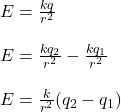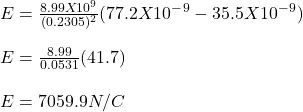Question

Find the magnitude of the electric field at a point midway between two charges +35.5 × 10−9 C and +77.2 × 10−9 C separated by a distance of 46.1 cm. The value of the Coulomb constant is 8.99 × 109 N · m2 /C 2 . Answer in units of N/C.

1.The electric field at the midway between two charges is 7059.9N/C

Explanation:

Given-

charge, q1 = 35.5 X 10⁻⁹ C

q2 = 77.2 X 10⁻⁹C

distance, r = 46.1cm

midway distance, r = 23.05 cm = 0.2305 m

Coulomb’s constant, k = 8.99 X 10⁹ Nm²/C²

Electric field, E = ?

We know,Therefore, electric field at the midway between two charges is 7059.9N/C